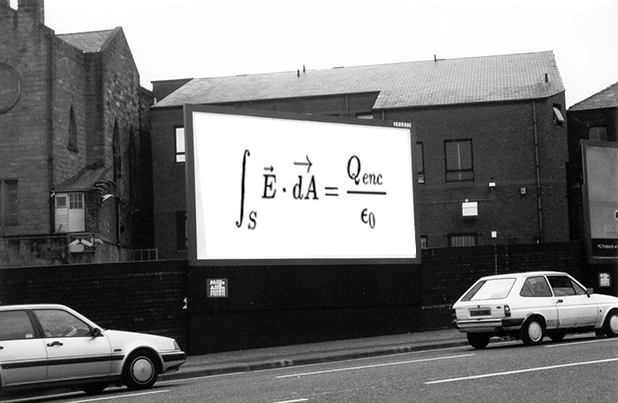# Can the Prince save you now?Gauss' law is a very powerful method to determine the electric field due to a distribution of charges. It can, for instance, be used to find the electric field due to an infinite plate. However, it is usually useless whenever there is a non-uniform field, i.e. there is broken symmetry.

One such case is that of a finite rectangular plate. Find the electric field due to a uniformly charged rectangular plate at a point 1 m above its center of mass.

Details And Assumptions

• Length = $\sqrt{\dfrac{13}{8}}\text{m}$
• Breadth = 3 m
• Total Charge on plate = $8 \times 10^{-8} \text{C}$
• $k=9 \times 10^9 \frac{\text{Nm}^2}{\text{C}^2}$
• Plate is an Insulator
###### This is an original problem.
×

Problem Loading...

Note Loading...

Set Loading...